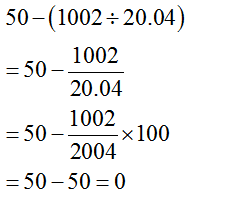• This is an assessment test.
• These tests focus on the basics of Maths and are meant to indicate your preparation level for the subject.
• Kindly take the tests in this series with a pre-defined schedule.

## Basic Maths: Test 15

Congratulations - you have completed Basic Maths: Test 15. You scored %%SCORE%% out of %%TOTAL%%. You correct answer percentage: %%PERCENTAGE%% . Your performance has been rated as %%RATING%%
 Question 1
$\displaystyle If\,\frac{50}{p}=\frac{p}{12\frac{1}{2}}$ then the value of p is:
 A $\displaystyle \frac{25}{2}$ B $\displaystyle \frac{4}{25}$ C $\displaystyle 4$ D $\displaystyle 25$
Question 1 Explanation:
$\displaystyle \begin{array}{l}\,\frac{50}{p}=\frac{p}{12\frac{1}{2}}\\\Rightarrow \frac{50}{p}=\frac{2p}{25}\\\Rightarrow 2{{p}^{2}}=50\times 25\\\Rightarrow 2{{p}^{2}}=1250\\Therefore\,\,p=25\end{array}$
 Question 2
$\displaystyle \frac{{{\left( 6.25 \right)}^{\frac{1}{2}}}\times {{\left( 0.0144 \right)}^{\frac{1}{2}}}+3}{{{\left( 0.027 \right)}^{\frac{1}{3}}}\times {{\left( 81 \right)}^{\frac{1}{4}}}}$
 A 0.14 B 1.46 C 1.36 D 3.6
Question 2 Explanation:
$\displaystyle \begin{array}{l}\frac{{{\left( 6.25 \right)}^{\frac{1}{2}}}\times {{\left( 0.0144 \right)}^{\frac{1}{2}}}+3}{{{\left( 0.027 \right)}^{\frac{1}{3}}}\times {{\left( 81 \right)}^{\frac{1}{4}}}}\\=\frac{2.5\times 0.12+3}{0.3\times 3}=\frac{0.3+3}{0.9}=\frac{3.3}{0.9}=3.6\end{array}$
 Question 3
The value of $\displaystyle \frac{8}{9}\times \frac{3}{\frac{5}{6}\div \frac{2}{3}\,\,of\,1\frac{1}{4}}$
 A $\displaystyle \frac{8}{3}$ B $\displaystyle 1$ C $\displaystyle \frac{1}{2}$ D $\displaystyle \frac{2}{3}$
Question 3 Explanation:
$\displaystyle \begin{array}{l}\frac{8}{9}\times \frac{3}{\frac{5}{6}\div \frac{2}{3}\,\,of\,1\frac{1}{4}}\\=\frac{8}{9}\times \frac{3}{\frac{5}{6}\div \frac{2}{3}\,\,of\,\frac{5}{4}}\\=\frac{8}{9}\times \frac{3}{\frac{5}{6}\div \frac{10}{12}\,\,}\\=\frac{8}{9}\times \frac{3}{\frac{5}{6}\times \frac{12}{10}\,\,}=\frac{8}{9}\times \frac{3}{1}=8/3\end{array}$
 Question 4
$\displaystyle 4-\left[ 5-\left\{ 2+\left( -5+6-2 \right)2 \right\} \right]$ is equal to
 A -1 B 2 C 0 D -2
Question 4 Explanation:
$\displaystyle \begin{array}{l}p=4-\left[ 5-\left\{ 2+\left( -1 \right)2 \right\} \right]\\=4\times \left[ 5-\left\{ 2-2 \right\} \right]\\=4-\left[ 5-0 \right]\\=4-5=-1\end{array}$
 Question 5
On simplification, 50−(1002/20.04) is equal to:
 A 329 B 0 C 2993 D 2543
Question 5 Explanation:Once you are finished, click the button below. Any items you have not completed will be marked incorrect.
There are 5 questions to complete.
 ← List →